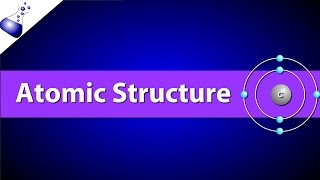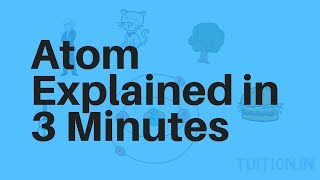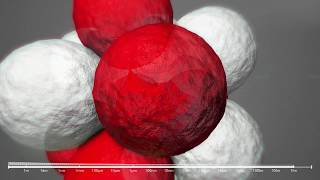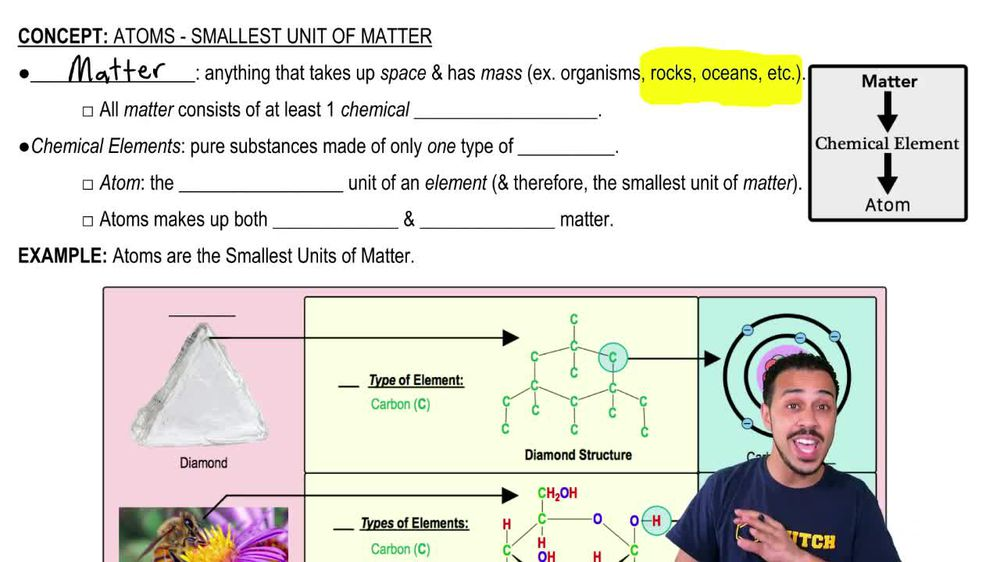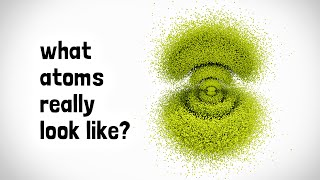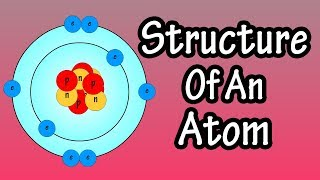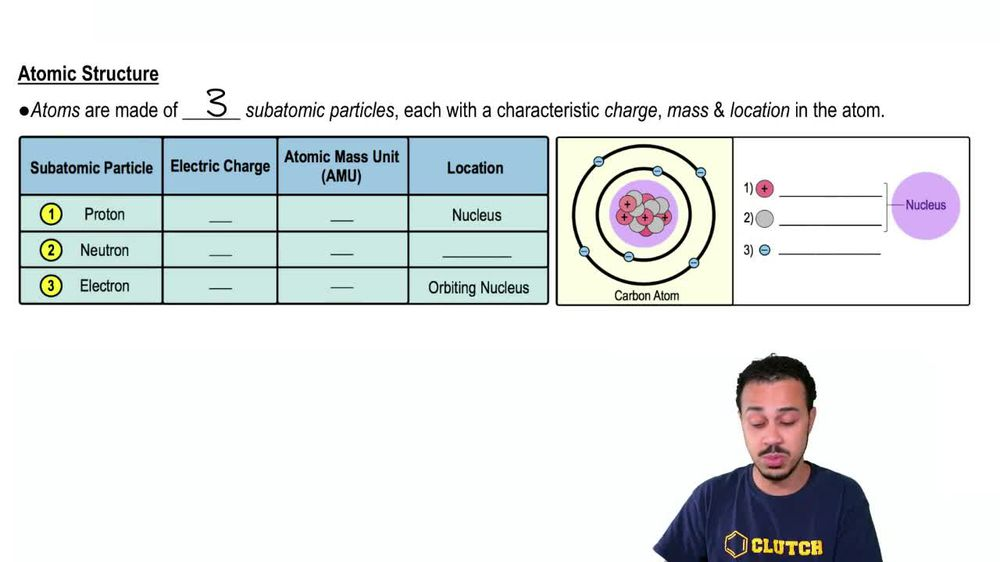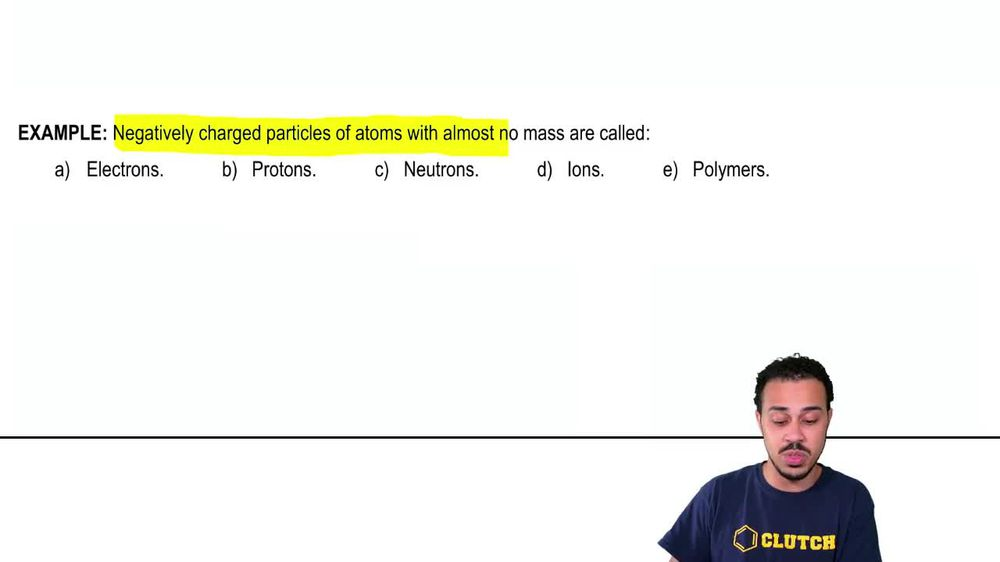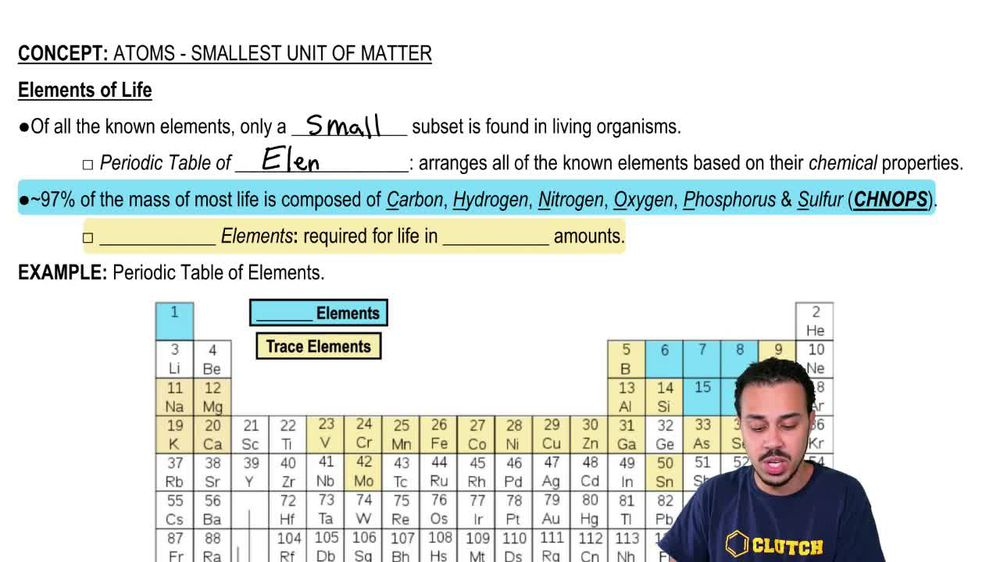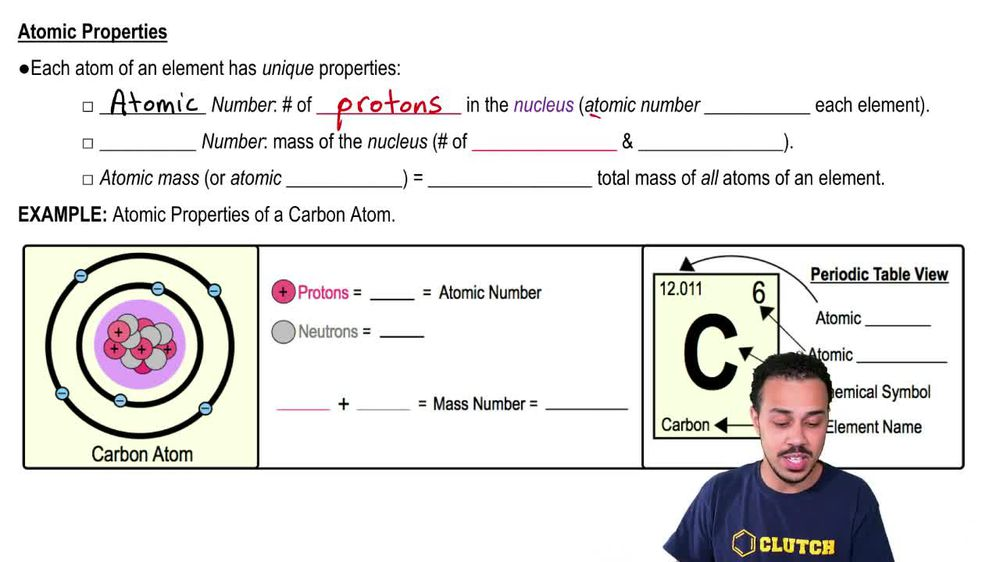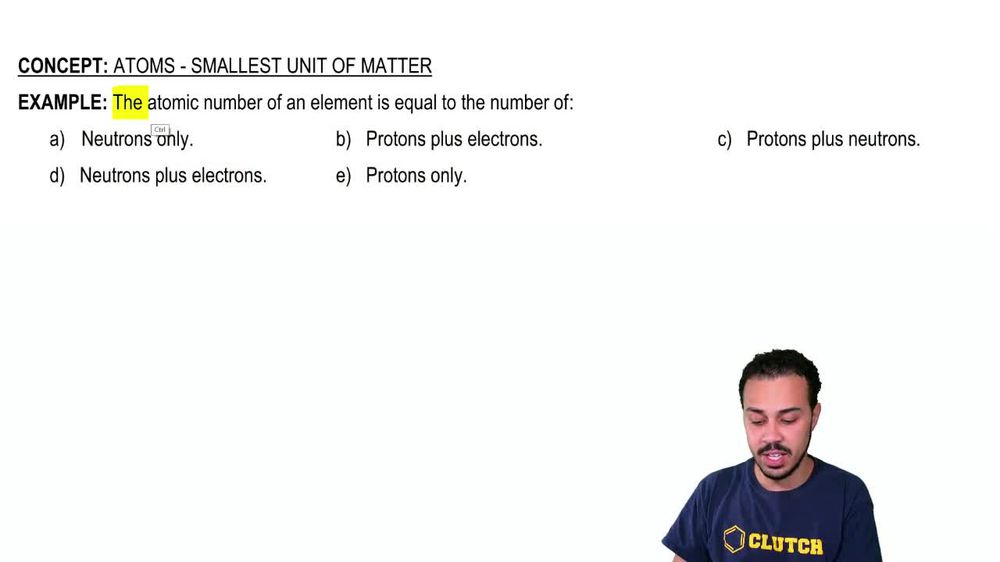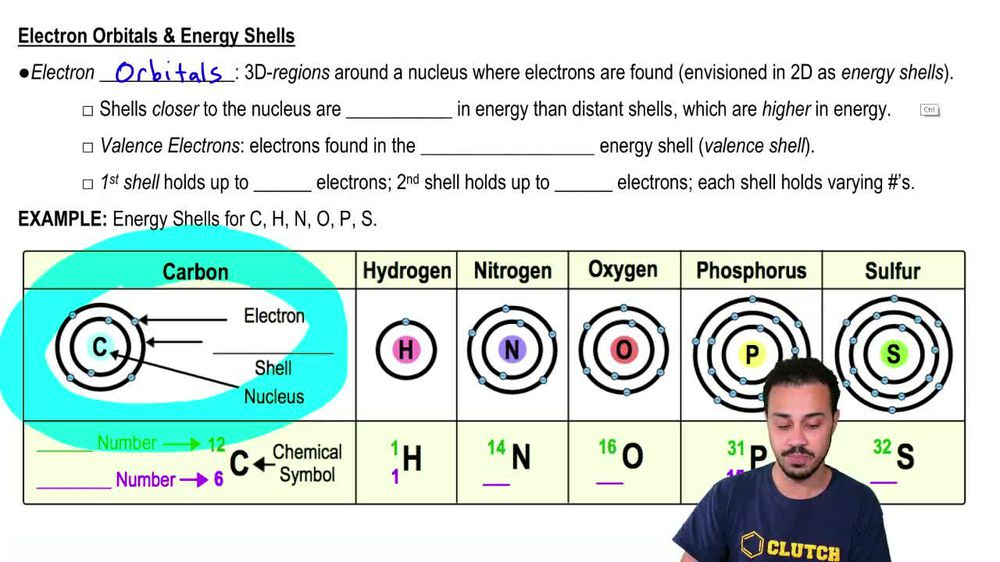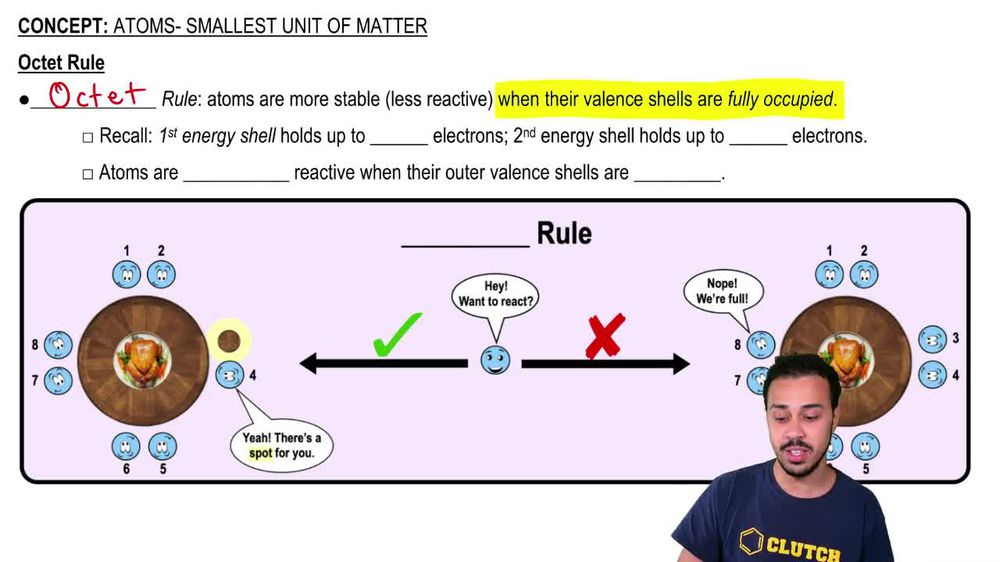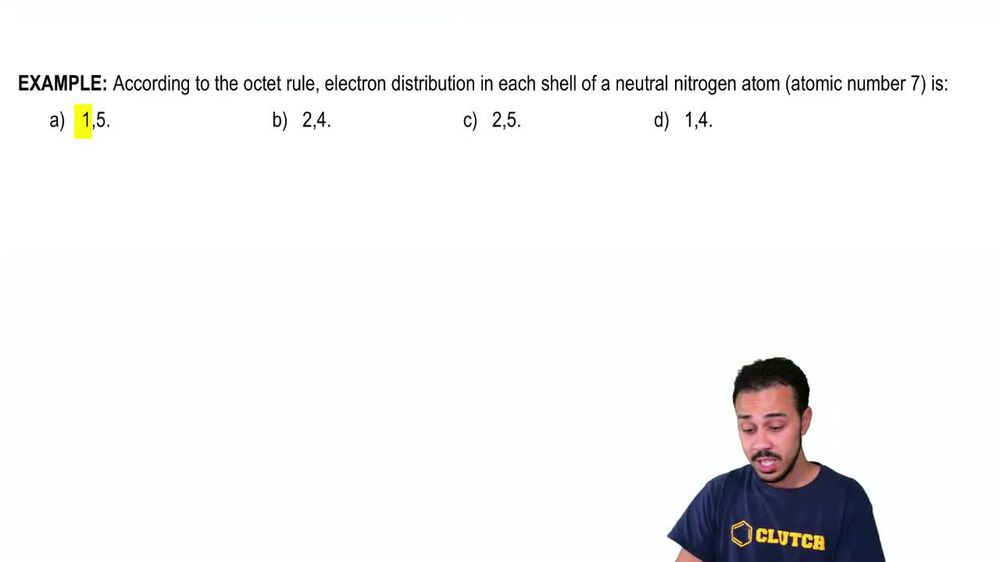Start typing, then use the up and down arrows to select an option from the list.
1. 2. Chemistry2. Atoms- Smallest Unit of Matter# Electron Orbitals & Energy Shells

by Jason Amores Sumpter
390 views
8
8
in this video, we're going to continue to talk about Adams by focusing on their electron, orbital's and energy shells. Now, electron orbital's are really defined as three dimensional regions or three D regions around the nucleus of an atom where electrons can be found now. Although electron orbital's are three dimensional regions, they can still be envisioned in two dimensions or in two D. As energy shells and really an hour course were mainly going to focus on the energy shell aspect, you'll learn Maura about the three dimensional shapes of electron orbital's in a chemistry class. So let's take a look down below at our example image here of the carbon atom on the far left to get a better understanding of these idea of this idea of energy shells. And so, which will notice, is that the chemical symbol here, right in the middle, represents the nucleus of this carbon atom, but then revolving around the nucleus of the carbon atom. What we have are these black circles, two of them, for that matter that represent energy shells. And once again, energy shells are really just the two D representation of the electron orbital's, which are really three dimensional regions. And so the energy shells once again are going to contain electrons. And so these little circles blue circles that you see around here are the electrons that are revolving or orbiting around the nucleus of the atom in these energy shells. Now, once again, any typical Adam could have multiple energy shells, as you can see from our image down below. And so if we focus in on the carbon atom here, notice that it has to energy shells once again has the one that is right here that is closer to the nucleus. And then it has a second energy shell that is further away from the nucleus. And so it's important to note is that the energy shelves that are closer to the nucleus of the atom are actually going to be lower and energy than the energy shells that arm or distant from the nucleus. And so, of course, this means that the distant shells that air further away from the nucleus are going to be higher and energy. And so because these distant shells are higher and energy, those are the ones that are mawr reactive, and so those are the ones that scientists tend to focus most of their attention on when they're looking at chemical bonds, which we'll talk about chemical bonds mawr later in a different video. But when we take a look at this carbon atom down below, notice once again that the energy shell that is furthest away from the nucleus is gonna be the one that is highest and energy, and it's gonna be the one that is more reactive and the one that tends to form chemical bonds. Now this leads us to this idea of valence electrons, because valence electrons are defined as the electrons that are found in the outermost energy shell, basically in the energy shell that is furthest away from the nucleus and the energy show that is furthest away from the nucleus. We can also call the Valence shell, And so the valence shell for carbon would be this one that is for this, away from the nucleus and electrons that are found within the valence shell. Like these four that we see here are termed valence electrons. And those were the once again the ones that are higher and energy and the ones that arm or reactive and so the other. Electrons that are closer to the nucleus are not as high and energy, and they're not nearly as reactive. And so they're not going to be the ones to form chemical bonds with other atoms. So that's important to keep in mind. Now, if you take a look down below the Adam, what you'll find is a different way to represent these atoms. And so you can see that the chemical symbol is gonna be shown here and then up to the top left of the chemical symbol. What we can show is the mass number which recall from our previous lesson videos, is just the total number of protons and neutrons and then to the bottom left of the chemical element. What we can show is the atomic number, which recall from our previous lesson videos, is the total number of protons in the nucleus. And so you can see that, uh, the carbon atom here has six protons in its nucleus which aren't being shown. They're just being symbolized here with the letter C, but then it also has six electrons that are negatively charged and balance out the positively charged protons. And so that means that the carbon atom that's being shown here is going to have a neutral net charge because the number of protons, which again is equal to six, balances out with the number of electrons, which is also equal to six when we count them up here. And so it has a neutral net charge and in fact all of the atoms that we're showing you right here are all going to have a neutral net charge as shown. So that's something important. Also keep in mind now, in terms of the first energy shell, the first energy shell is always going to hold a maximum of two electrons. The second energy shell is going to hold a maximum of eight electrons and then each of the shells that are beyond that are all going to hold a varying number of ah characteristic number of electrons and really, for our purposes, in this biology course, you really only need to know. The first electron holds up to two electrons and the second shell holds up to eight electrons. Sorry, The first shell holds up to two electrons, and the second shell holds up to eight electrons. So if we take a look down below the carbon atom once again notice that the first energy show here is holding a maximum of two electrons. And any additional electrons need to be in a different energy shell. And so this is going to be how we can represent the carbon atom with its energy shells like this. If we move on to the hydrogen atom right here, what you'll see is that its atomic number is one meaning it has one proton on its mass number appears also one which means that it only has one proton and no neutrons. And of course, because all of the atoms that we're showing you here are neutral, uh, have a neutral charge. The number of protons is gonna bounce out exactly what the number of electrons for all of the ones that are shown right here. And so if it has one proton, that means it also has one electron. And because it only has one electron, it only needs one energy shell. And so this is a way to represent the hydrogen atom now moving on to the nitrogen atom. Nitrogen atoms have seven protons in their nucleus. And so when we count up the number of electrons. You'll see that there are also seven electrons to balance out the positively charged protons. And so, for its energy shell noticed that it gets filled. The first energy shell gets filled with two electrons, and then all of the other electrons need to make their way into the third. Um, sorry, the second shell, which holds up to a maximum of eight electrons. Um, now, if we move on to the oxygen atoms, Oxygen has a characteristic atomic number of eight, and so its mass number is 16, which means it has eight neutrons in its nucleus. But once again you can count up the total number of electrons it's going. It's going to add up to the total number of protons if it's a neutral oxygen atom, which once again these are all neutral atoms. And so, which will see once again, is that the first two electrons are gonna go into the first energy shell, and then all other electrons are gonna move to the second shell, which can hold up to a maximum of eight electrons. And here it holds six electron, so it's fine. However, once we go to the phosphorus atom over here. Uh, notice that its atomic number is 15, meaning it has 15 protons in its nucleus. It's mass numbers 31 meaning it has 16 neutrons in its nucleus. But once again, because these air neutral atoms, the number of protons here eyes gonna equal the number of electrons. So when you count up all of the electrons here, you'll count that there are 15 of them. Once again, the first energy shell is filled with two electrons. Eso then it goes on to the second shell, and the second shell is filled with eight electrons. So that means that all of the other electrons need to move into the third shell here. Um and, uh, the sulfur atom over here is a similar example. It it's going to have a atomic number of Ah, mass number of 32 which means it has 16 neutrons in its nucleus. And then, of course, the number of electrons that you see revolving around the nucleus is going to match the number of protons if it's a neutral atom. And once again the first shell gets filled with two electrons, the second shell gets filled with eight electrons and all of the other electrons gonna need to move into the third shell here for this sulfur. And so this year really concludes our lesson on electron, orbital's and energy shells, and we'll be able to get some practice applying these concepts moving forward in our next few videos, so I'll see you in our next one.